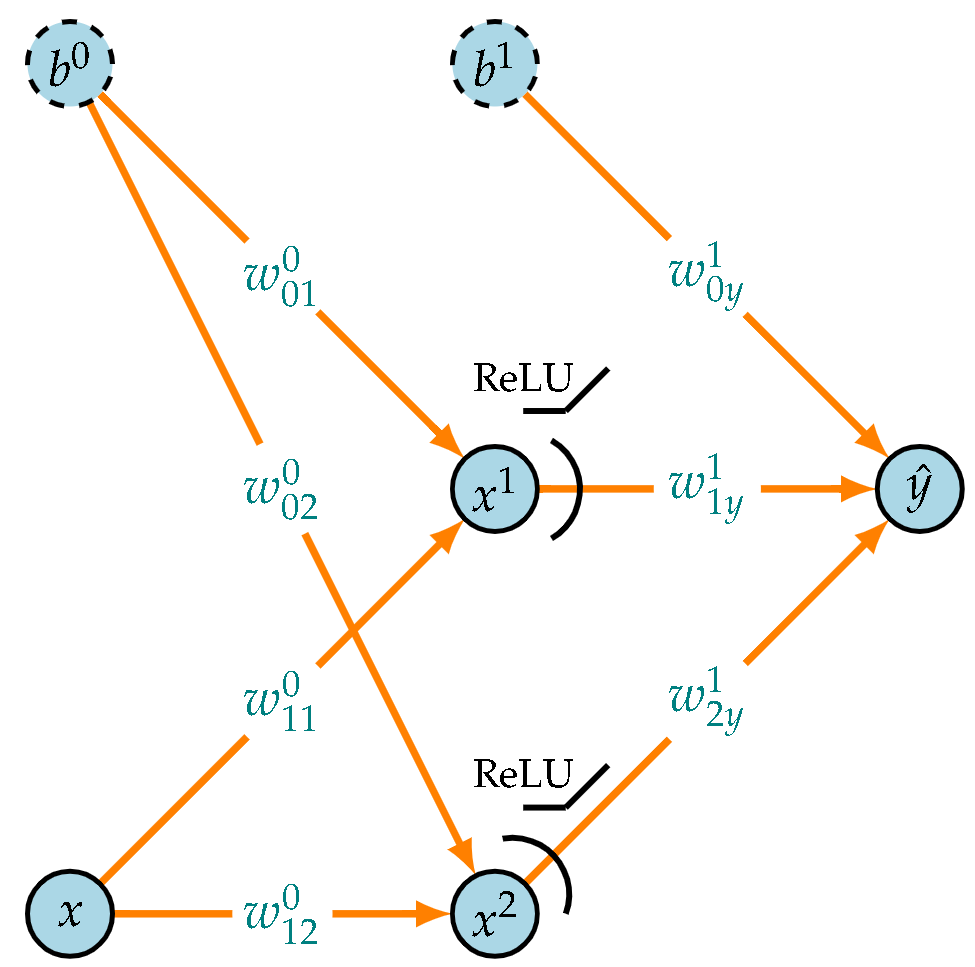# Visualizing function approximation using dense neural networks in 1D, Part I# Approximations with Neural networks

Modern libraries like TensorFlow, Pytorch, Theano, etc., have made it extremely easy to define and train Neural Networks. However, there is a (growing) list of things that one needs to consider for a proper definition and training of a NN. The worse thing is that the default values usually won’t work. We’ll delve deeper into this in Part II. For now, we’ll concentrate in solving the problem at hand.

## An initial model

We’ll begin with the most basic form of a neural network, which consists of a single output unit with no hidden layer.

It has only 2 parameters and the prediction (ŷ) is simply a linear combination of the input(x) and the bias(b) via the weights(w).

It is not surprising at all that the answer is very close to the one obtained with sklearn (prediction = -0.677 + 2.03x) . In fact, the fit with sklearn was computed exactly while this one is just an approximation of the minimum of the loss function obtained with gradient descent.

Nevertheless, it is usually a good idea to have a simple and trustworthy baseline model to make sure that everything is working as intended, specially regarding data loading, loss computations and the optimization method. Furthermore, this is a convex problem and as such, it has an easily obtainable unique solution for which the loss is minimal.

# One hidden unit

We’ll start adding complexity to the model and the first nonlinearity in the form of a ReLU function. In the end though, the approximation is simply in the shape of a single ReLU function which is only a slight improvement in complexity compared to a single line. Namely,

The results is that the optimization simply chooses to ignore that extra complexity that ReLU provides and the solution coincides with the previous one, as seen in the next figure.

# Two hidden units

Things get interesting when a second hidden unit is introduced and the total number of parameters increases to 7.

In this case there are two ReLU components available to approximate the function and, as the solution shows, they both contribute in lowering the loss. The formula below provides a way to graphically split the solution into its two ReLU components and the constant bias term.

In this case the minimization of the loss arrives at a point where the two ReLU components along with the bias terms work together to provide a good fit to the data. The algorithm decided fto provide a better fit to the left and center data points, since a constant approximation at the right side does not result in a large MSE.

To be continued in Part II…

• 3 hidden units
• An increasing number of parameters
• Networks with 2 hidden layers
• Key takeaways from this exercise